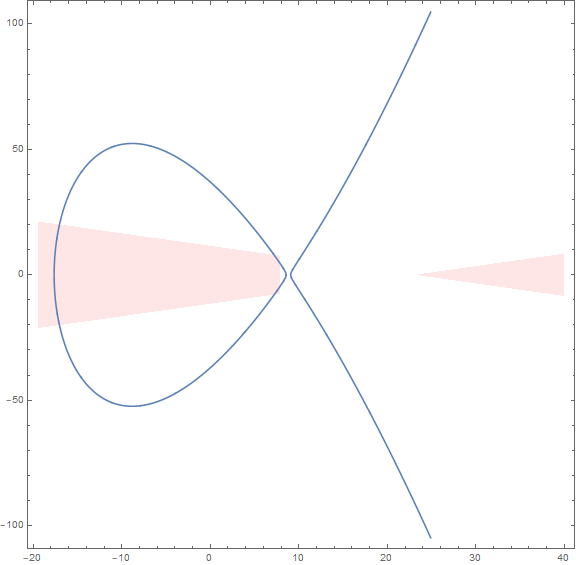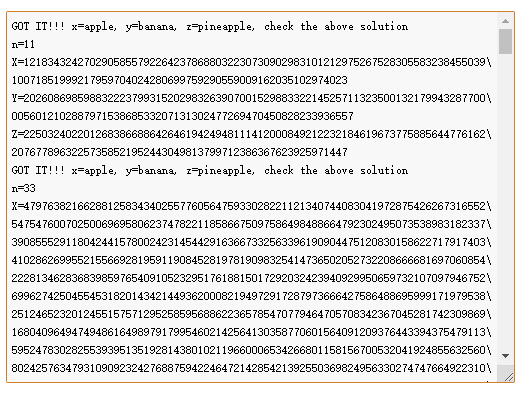# cube

## 解题前的阿巴阿巴C := Curve(P2,x^3 - 3*x^2*y - 3*x^2*z - 3*x*y^2 - 5*x*y*z - 3*x*z^2 + y^3 - 3*y^2*z - 3*y*z^2 + z^3);

E,f := EllipticCurve(C);

## 解题过程## 说在最后？？？？？我丢你妈的，原题，就尼玛离谱，合着我看了半天，，，，啥也不是，草。

$x = 4(n+3)(a+b+2c)/(c-(n+2)(a+b))$

$y = (8n^2+44n+60)(a-b)/(c-(n+2)(a+b))$

$a = \frac{8(N+3)-x+y}{2(N+3)(4-x)}$

$b = \frac{8(N+3)-x-y}{2(N+3)(4-x)}$

$c = \frac{-4(N+3)-(N+2)x}{(N+3)(4-X)}$

# re

×